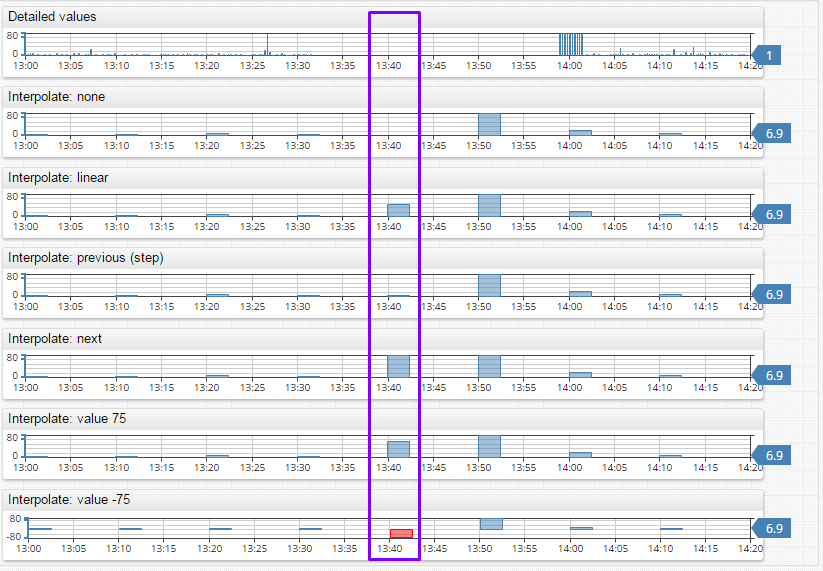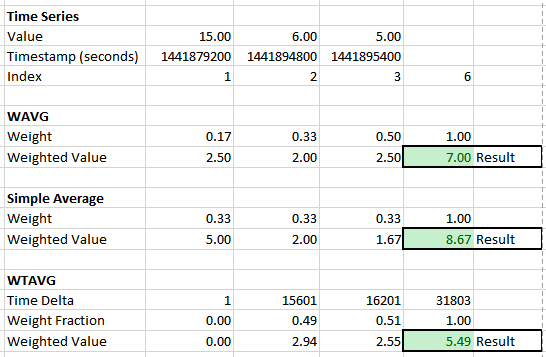# Aggregators

NameDescription
`count`Number of samples during the period.
`min`Minimum value during the period.
`max`Maximum value during the period.
`sum`Sum of values during the period.
`avg`Average value during the period.
`percentile_999`
`percentile_995`
`percentile_99`
`percentile_95`
`percentile_90`
`percentile_75`
`percentile_50`
Percentile(N) is a number which is greater than the N percentage of samples in the period.
For example, `percentile_995` (99.5%) represents a number greater than 99.5% of all observations received during the period. The remaining 0.5% of all observations are greater than `percentile_995`.
Percentile argument can be any number between 0 and 100, for example: `percentile_25`.
The NIST (National Institute of Standards and Technology) method is used to calculate percentiles.
`median``percentile_50`
`standard_deviation`Standard deviation of values during the period, `square root of the variance`.
`first`First value received during the period.
`last`Last value received during the period.
`delta`Delta is computed as the difference between the last value in the period and the last value in the previous period. If no last value is present in the previous period, then `delta` is calculated as the difference between the last and first values during the current period. See also `counter` aggregator.
`counter`Returns sum of the differences between consecutive values in the period. If the difference between any two values is negative, the difference is replaced with the value itself. If the difference between values is always non-negative, the `counter` aggregator will return the same value as the `delta` aggregator. Example explaining difference between `COUNTER` and `DELTA`: View
`wtavg`An average resulting from the multiplication of each component by a factor reflecting its importance.
Weight is assigned based on timestamps rather than index.
Data points with older timestamps contribute significantly less to the weighted mean than data points with more recent timestamps.
The weight of a sample is proportional to the current_time – first_time + 1 (in seconds), where current_time is the timestamp of the sample, and first_time is the timestamp of the first sample received during the period.
The weights are normalized so that their sum is equal to 1.
See calculation example below.
`wavg`A weighted average of samples received during the period.
The weight of a sample is proportional to the index of the sample in the time-ordered array of all samples during the period.
The weights are normalized so that their sum is equal to 1.
A weighted average is sum of the sample’s values multiplied by their weights.
See a calculation example below.
`min_value_time`Time when the minimum value (`min`) occurred during the period.
`max_value_time`Time when the maximum value (`max`) occurred during the period.
`threshold_count`Number of threshold violations during the period.
A violation is a sequence of one or more successive data points exceeding the specified threshold.
Example: the following values are collected every 5 minutes: `1, 2, 5, 7, 1, 1`
If the threshold is set to 4, then one sequence (5,7) exceeds the threshold.
`threshold_duration`Total duration of threshold violations during the period, in milliseconds.
A violation is a sequence of one or more successive data points exceeding the specified threshold.
Example: the following values are collected every 5 minutes: `1, 2, 5, 7, 1, 1`
If the threshold is set to 4, then one sequence (5,7) exceeded the threshold and its total duration based on linear interpolation is 550 seconds. If the threshold is 5, then duration is 850 seconds.
For periods at the beginning of the timespan, at the end of the timespan or after a missing period, the value at the beginning of the period is set to the first value, and the value at the end of the period is set to last value.
`threshold_percent`Percent of time during which the value was below threshold (no violation).
Formula: `threshold_percent = 100 - threshold_duration / period_duration * 100`

#### Interpolation Functions

If the period doesn’t contain any detailed values, it will not be included in the results. Aggregator behavior can be modified by specifying an interpolation function.

FunctionDescription
NONE (default)No interpolation. Periods without any raw values are excluded from results.
LINEARCalculate period value using linear interpolation between previous and next period values.
PREVIOUSSet value for the period based on the previous period’s value.
NEXTSet value for the period based on the next period’s value.
VALUESet value for the period to a specific number.

Interpolation Examples:View in Chartlab

#### AVG, WAVG and WTAVG Example`wtavg()` – weighted time average.

Calculation: `Weight = (sample.time - first.time)/(last.time - first.time + 1)`

#### Aggregation Example

TimestampValueAggregators
2015-09-10 10:00:0011`first = 11`
`delta = 0`
2015-09-10 11:00:009`min = 9 | min_value_time = 2015-09-10 11:00:00`
`delta = -2`
2015-09-10 12:00:0014`delta = 5`
2015-09-10 13:00:0019`delta = 5`
2015-09-10 14:00:0021`max = 21 | max_value_time = 2015-09-10 14:00:00`
`delta = 2`
2015-09-10 15:00:0015`delta = -6`
2015-09-10 16:00:0014`delta = -1`
2015-09-10 17:00:0011`delta = -3`
2015-09-10 18:00:0016`delta = 5`
2015-09-10 19:00:0014`delta = -2`
2015-09-10 20:00:0013`last = 13`
`delta = -1`

Aggregators:
Threshold is set to `20`
Period is set to `11 hours`, so it includes with whole data set.

NOTE: Period `starttime` is inclusive and `endtime` is exclusive.
Meaning that `startime = 2015-09-14 10:00:00` will include data points that occurred exactly at `10:00:00` and later.
`Endtime = 2015-09-14 11:00:00` will include data points that occurred up to `10:59:59`, excluding points that occurred at `11:00:00` and later.

```count = 11 first = 11 last = 13 min = 9 min_value_time = 2015-09-10 11:00:00 max = 21 max_value_time = 2015-09-10 14:00:00 sum = 157 avg = 14.27 percentile_999 = 21 percentile_995 = 21 percentile_99 = 21 percentile_95 = 21 percentile_90 = 20.6 percentile_75 = 16 percentile_50 (median) = 14 standard_deviation = 3.47 wavg = 14.47 wtavg = 14.51 threshold_count = 1 threshold_duration = 3600000 threshold_percent = 88.9```
##### Chart Lab Examples:

Box Chart illustrating the percentiles: view

Histogram illustrating the percentiles: view

Time Chart illustrating delta: view

Time Chart comparing counter and delta aggregators: view